# Journal Club: Chen et al, 2018: Neural Ordinary Differential Equations

In the 2018 NeurIPS conference, 4,845 papers were submitted. The paper I’m reviewing here by Chen et al, 2018, titled Neural Ordinary Differential Equations, won best paper award. The paper discusses using continuous Ordinary Differential Equations (ODE) for Neural Networks (NN) as opposed to the sorts of discrete layers used in the standard Recurrent Neural Networks (RNN).

## Introduction

Recurrent Neural Networks (RNNs) use an iterative process where the output of one pass is used as the input for the next:Basic Neural Network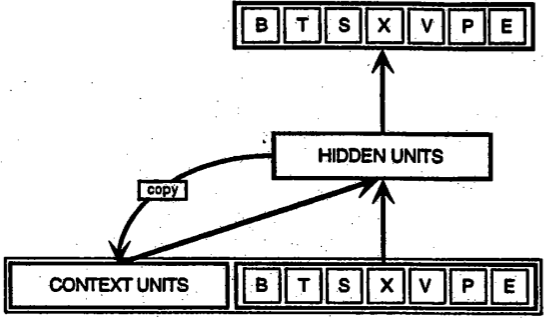Recurrent Neural Network (RNN) showing that the output for a time step t is sent back to be used as the input in the next iteration. This information is called hidden because it is not output, making the neural network a black box (the user doesn’t actually know what occurred ‘inside’).

The paper explains that residual and recurrent neural networks use discrete approximations of continuous transformations described by the equation:Where, hₜ is the “hidden” information at time step t, and f(hₜ, θₜ) is the learned function of the current hidden information and parameters θₜ. The parameters, θₜ, are the weights and biases applied to each unit in each discrete layer. For a great tutorial on how Neural Networks work, I suggest the video series on it by 3Blue1Brown on Youtube:

The paper’s author’s Ordinary Differential Equations (I’ll call it ODE), however, treat the layers like a continuous function, making them more like a vector field than. Thinking of the unit states like a vector field is exactly how the author’s of this paper thought about it, such that calculating the weighted state of a unit is like doing a linear transformation of a vector. As the video above describes, the weights can be treated like a matrix performing a transformation on the vector, which represents the connection strength between units. The connection strengths can then be pictured as a vector, with the ODE model being a vector field as opposed to discrete vectors: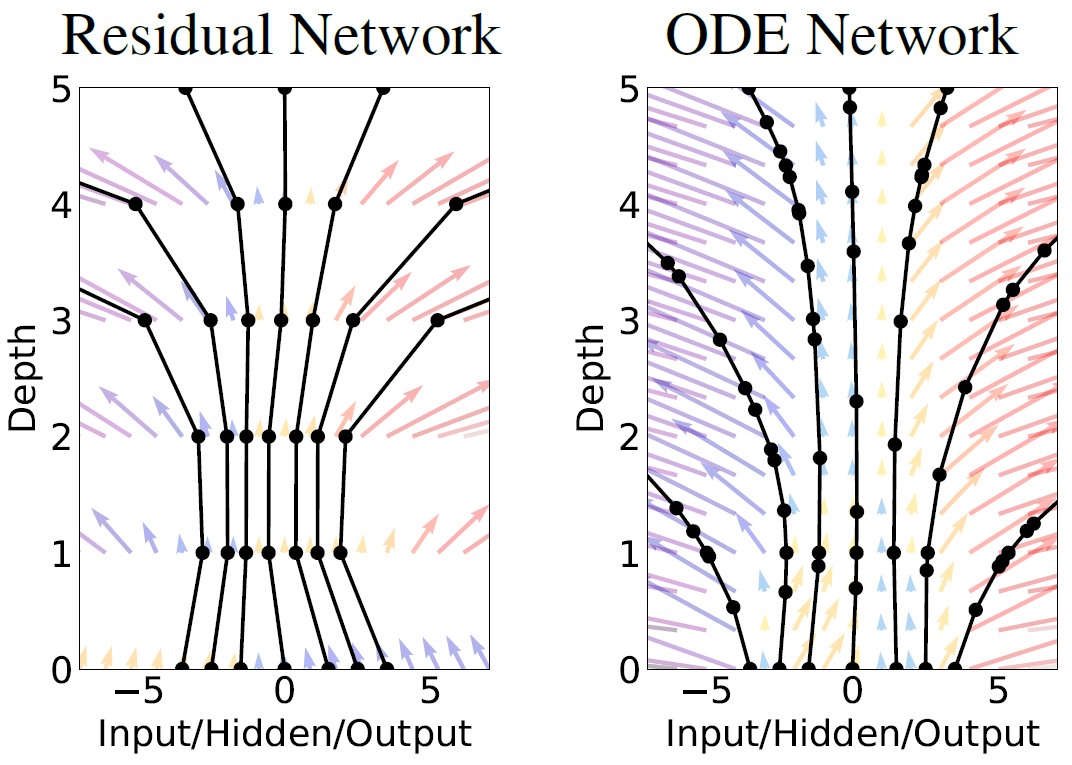Left: in an RNN, the vectors are discrete for each layer. Right: with the ODE network, there are no discrete layers and the unit values behave like a vector field. The strength of each unit connection changes continuously through the network.

The change in the hidden information with respect to time is described by the ODE: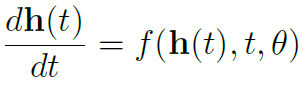This increases accuracy for the time series predictions while decreasing memory usage.

## Backpropagation of ODE Solutions

NNs calculate the error in their prediction from each iteration using what is called gradient descent. This is used for training the NN on a given set of data.Calculating the Cost, C, or the ‘wrongness’ of the NN’s output. The average cost over the training data set is a measure of how bad the NN is.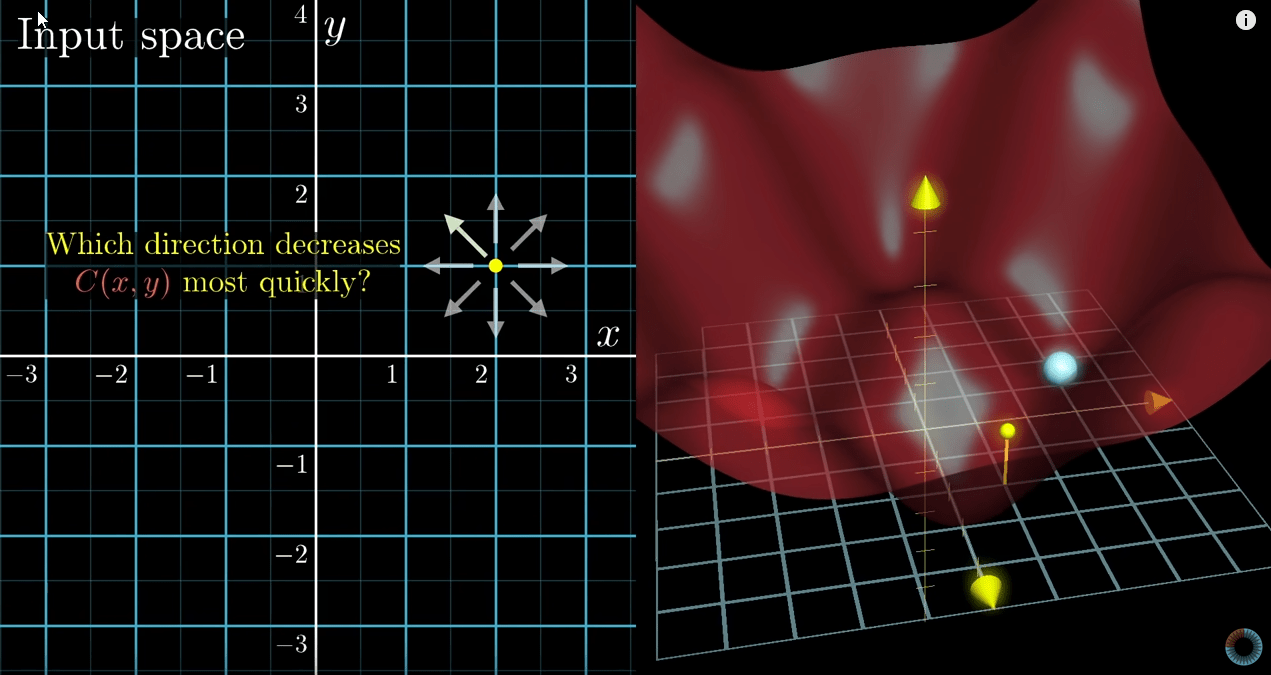To train the network, you need to find where the C is lowest by locating the minimum in a gradient field of the C. The lowest C will change the weights such that the NN will give the best outputs for the data set.

For a better understanding, watch the 3Blue1Brown video (where the above two screenshots were taken):

Backpropagation is what Neural Networks use to better fit the data at each unit. It’s how the weights are adjusted after C is calculated. Using some complicated algorithms, the contribution of each unit to C can be calculated such that the weight of the unit can be adjusted to lower C. Once again, 3Blue1Brown does a fantastic job of explaining:

To solve an ODE, we normally use integration using the Euler method (or related methods). The Euler method calculates the shape of an unknown curve which starts at a given point and satisfies a given differential equation. While the curve is unknown, its starting point A0 is known. From the differential equation the slope at A0 can be computed. Move along that tangent line up to a point A1. Along this small step, the slope does not change too much, so A1 will be close to the curve. If we pretend that A1 is still on the curve, the same reasoning as for the point A0 above can be used. After several steps, a polygonal curve A0, A1, A2, A3, … is computed.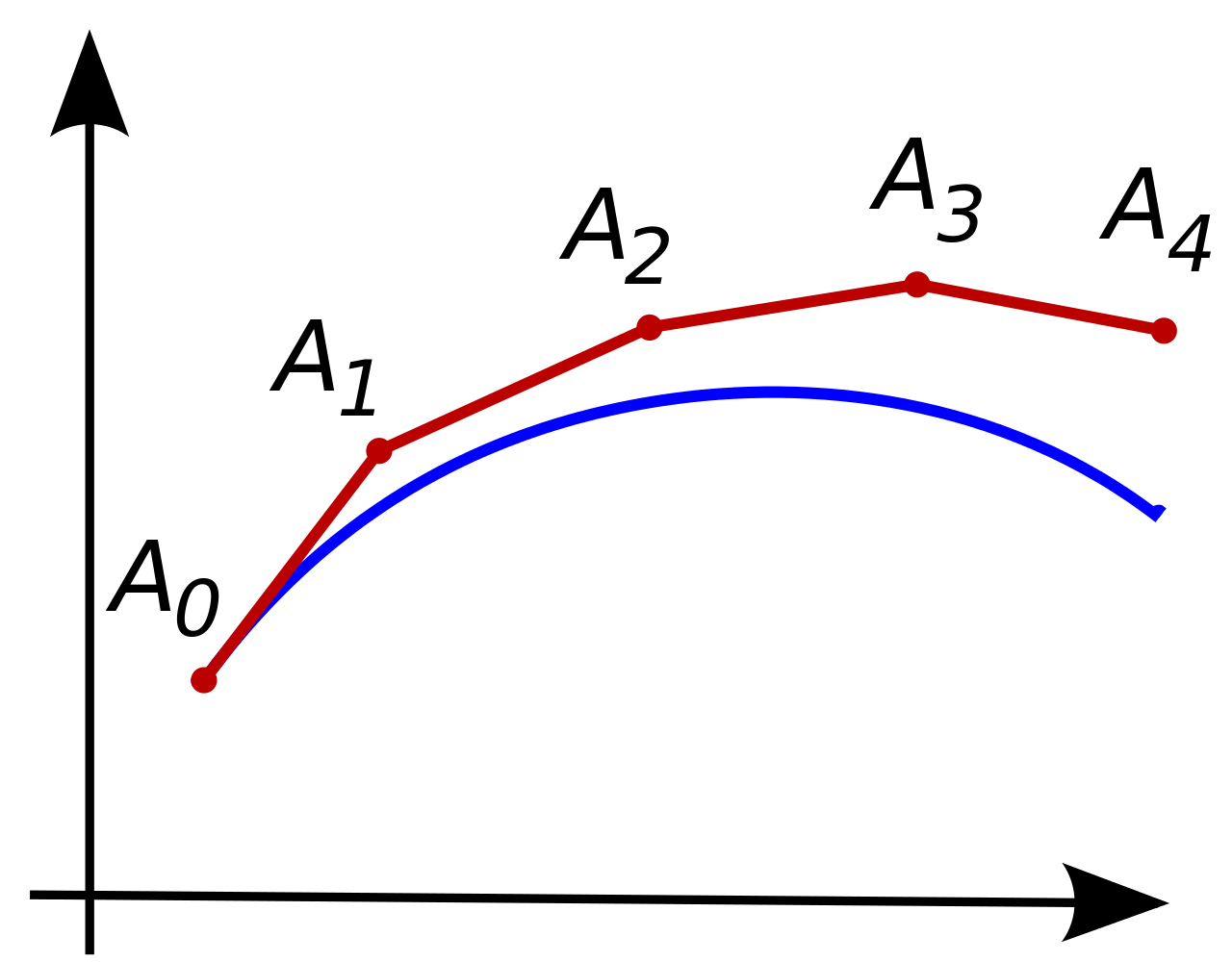This curve is an approximation of the actual curve, but the error between the two can be made small if the step size is small enough.

This is computationally inefficient and when differentiation is required of the integration steps to add up all the gradients of the network parameter for backpropagation it incurs a high memory cost. Instead, the authors use what is called the adjoint method. The specifics are a bit more complicated, save that they use a black box ODE solver that they call (drumroll) ODESolve. But the result is the same thing as the Euler method, though much more computationally efficient. With the gradients calculated, adjustments to the weights of the vector field can be made similar to backpropagation done in RNNs.

## Application of ODE Networks

The authors tested their method using supervised learning. The aim was that with fewer parameters involved, ODESolve can provide the same precision as a Residual Network (ResNet). A single block of ODEsolver was compared with a ResNet with six layers (and a couple other types of RNN). What they found is that the number of paremeters was three times fewer than ResNet while having about the same amount of error.The paper goes on to talk about continuous normalizing flows and generative latent function time-series models. I’m not going to get into these more complicated ways of using their method.

## Conclusion: What’s the Punchline Here?

This paper proposes a novel method for deep learning that could vastly improve the field in terms of precision and computational efficiency. Further research will need to be done to find any other limitations – and for other potential uses and advantages.